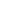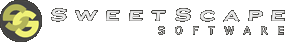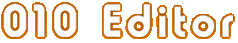Edit AnythingProfessional text and hex editingwith Binary Templates technology.010 Editor - Text/Hex Editor Homepage

 Hex Operations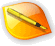The Hex Operations tool provides any easy way to apply mathematical operations to a set of bytes. Open the Hex Operations dialog by clicking the 'Tools > Hex Operations' menu option and selecting an operation from the list.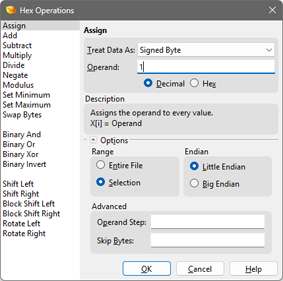All hex operations treat the bytes in the file as an array. Select the data type of the array by choosing a type from the Treat Data As drop-down list. Enter a number in the Operand field using any of the formats described in the Introduction to Number Systems. Note that the operand is assumed to be hex if the Hex toggle is set, or decimal if the Decimal toggle is set. How the Operand is applied to the data is dependant upon which operation is selected. The following list describes each operation in C notation, assuming that X[i] represents each value in the file to be modified. Assign: X[i] = Operand Add: X[i] += Operand (this is equivalent to X[i] = X[i] + Operand) Subtract: X[i] -= Operand (this is equivalent to X[i] = X[i] - Operand) Multiply: X[i] *= Operand (this is equivalent to X[i] = X[i] * Operand) Divide: X[i] /= Operand (this is equivalent to X[i] = X[i] / Operand) Negate: X[i] = -X[i] Modulus: X[i] = X[i] % Operand (the modulus operator % computes the remainder after dividing X[i] by the Operand) Set Minimum: Sets a minimum limit for X[i]. If X[i] is less than the Operand, X[i] is set to the Operand. Set Maximum: Sets a maximum limit for X[i]. If X[i] is greater than the Operand, X[i] is set to the Operand. Swap Bytes: Swap the bytes of X[i] Binary And: X[i] &= Operand Binary Or: X[i] |= Operand Binary Xor: X[i] ^= Operand Binary Invert: X[i] = ~X[i] Shift Left: X[i] <<= Operand Shift Right: X[i] >>= Operand Block Shift Left: Similar to Shift Left except data is treated as one long block. Bytes shifted off of X[i+1] will be shifted onto X[i]. Block Shift Right: Similar to Shift Right except data is treated as one long block. Bytes shifted off of X[i] will be shifted onto X[i+1]. Rotate Left: Similar to Shift Left except that bytes shifted off of X[i] will be added to the right side of X[i]. Rotate Right: Similar to Shift Right except that bytes shifted off of X[i] will be added to the left side of X[i]. Note that the Operand is not used for some operations and some operations can only be used on certain data types. A description of the selected operation is shown in the Description box and further options for the dialog can be controlled by clicking the Options button. If no bytes are selected in the file, the Operation will be applied to the whole file. If a selection is made, the Operation will be applied to the selected bytes if the Selection toggle is set, or the whole file if the Entire File toggle is set. By default, the data for the operation will be assumed to have the same endian as the file (see Introduction to Byte Ordering for more information). To change the endian of the operation, click the Little Endian or the Big Endian toggle. The Advanced box contains two fields: Operand Step and Skip Bytes. If a value is entered in Operand Step, that value will be added to the Operand after modifying each value in the file. The Operand Step can be used to easily perform a number of complex operations, including building arrays. For example, select 256 bytes in a file and perform an Assign operation on the bytes with an Operand of '0' and an Operand Step of '1'. The result will be an array with the values 0 up to 255. If a value is entered in the Skip Bytes field, that number of bytes will be skipped after each value is modified in the file. This feature can be used to skip over bytes that should not be modified. For example, if a binary file contains a series of employee records containing an integer ID number followed by a 40 character Name, the Skip Bytes field can be used to modify the ID number without modifying the Name. Use an Add operation on integers with '1' as the Operand and '40' as the Skip Bytes value. For more complex operations, scripts can be used. See Introduction to Templates and Scripts for more information.010 Editor v13.0 Manual - Windows EditionCopyright © 2003-2022 SweetScape Software - www.sweetscape.com

This is the manual for 010 Editor, a professional hex editor and text editor. Use 010 Editor to edit the individual bytes of any binary file, hard drive, or process on your machine. 010 Editor contains a whole host of powerful analysis and editing tools, plus Binary Templates technology that allows any binary format to be understood.Newsletter - Receive special offers, tips, tricks and news. Join now010 Editor v13.0.2 is here! What's new?NavigationProducts010 Editor